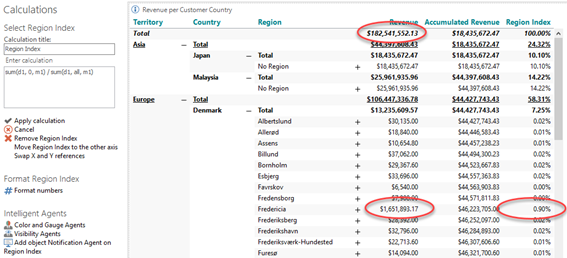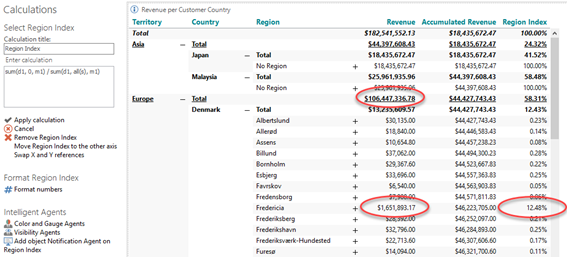# Siblings Modifier

• Expand all Countries (e.g. by use of the '+' in the upper right corner of the object).
• Add another calculation, Index per Region:
• sum(d1, 0, m1) / sum(d1, all, m1). Format as Percent.Note: When no Hierarchy Modifier has been applied, the profit of each Region will be calculated as a percentage of the equivalent of the grand total.
• Add the “siblings” modifier to the calculation:
• sum(d-1, 0, m1) % sum(d-1, all(s), m1).Note: By adding the “siblings” modifier, the profit of each Region will now be calculated as a percentage of the equivalent of the subtotals for each Country.